# Homology of compact orientable surfaces

This article describes the value (and the process used to compute it) of some homotopy invariant(s) for a topological space or family of topological spaces. The invariant is homology and the topological space/family is compact orientable surface
Get more specific information about compact orientable surface | Get more computations of homology

## Statement

Suppose$g$ is a nonnegative integer. We denote by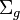$\Sigma_g$ the compact orientable surface of genus$g$, i.e.,$\Sigma_g$ is the connected sum of$g$ copies of the 2-torus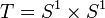$T = S^1 \times S^1$(and it is the 2-sphere$S^2$when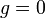$g = 0$). In other words,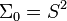$\Sigma_0 = S^2$,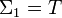$\Sigma_1 = T$,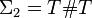$\Sigma_2 = T \# T$, and so on.

### Unreduced version over the integers

We have: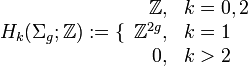$H_k(\Sigma_g;\mathbb{Z}) := \lbrace\begin{array}{rl} \mathbb{Z}, & k = 0,2 \\ \mathbb{Z}^{2g}, & k = 1 \\ 0, & k > 2\end{array}$

In other words, the zeroth and second homology groups are both free of rank one, and the first homology group is$\mathbb{Z}^{2g}$, i.e., the free abelian group of rank$2g$.

### Reduced version over the integers

We have:$\tilde{H}_k(\Sigma_g;\mathbb{Z}) := \lbrace\begin{array}{rl} 0, & k = 0 \\\mathbb{Z}^{2g}, & k = 1 \\ \mathbb{Z}, & k = 2 \\ 0, & k > 2 \end{array}$

### Unreduced version with coefficients in$M$

With coefficients in a module$M$ over a ring$R$, we have:$H_k(\Sigma_g;M) := \lbrace\begin{array}{rl} M, & k = 0,2 \\ M^{2g}, & k = 1 \\ 0, & k > 2\end{array}$

### Reduced version with coefficients in$M$$\tilde{H}_k(\Sigma_g;M) := \lbrace\begin{array}{rl} 0, & k = 0 \\ M^{2g}, & k = 1 \\ M, & k = 2 \\ 0, & k > 2 \end{array}$

## Related invariants

These are all invariants that can be computed in terms of the homology groups.

Invariant General description Description of value for genus$g$ surface Comment
Betti numbers The$k^{th}$ Betti number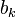$b_k$ is the rank of the torsion-free part of the$k^{th}$ homology group.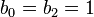$b_0 = b_2 = 1$,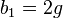$b_1 = 2g$, all higher$b_k$ are zero
Poincare polynomial Generating polynomial for Betti numbers$1 + 2gx + x^2$
Euler characteristic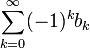$\sum_{k=0}^\infty (-1)^k b_k$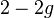$2 - 2g$ In particular, this means that the Euler characteristic is negative for$g > 1$, and also that the Euler characteristic and genus can be computed in terms of each other -- given the genus, we can find the Euler characteristic, and vice versa.

## Facts used in homology computation

1. Homology of spheres: This tackles the$g = 0$ case.
2. Homology of torus: This tackles the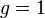$g = 1$ case.
3. Homology of connected sum: This tackles the inductive procedure of taking connected sums.